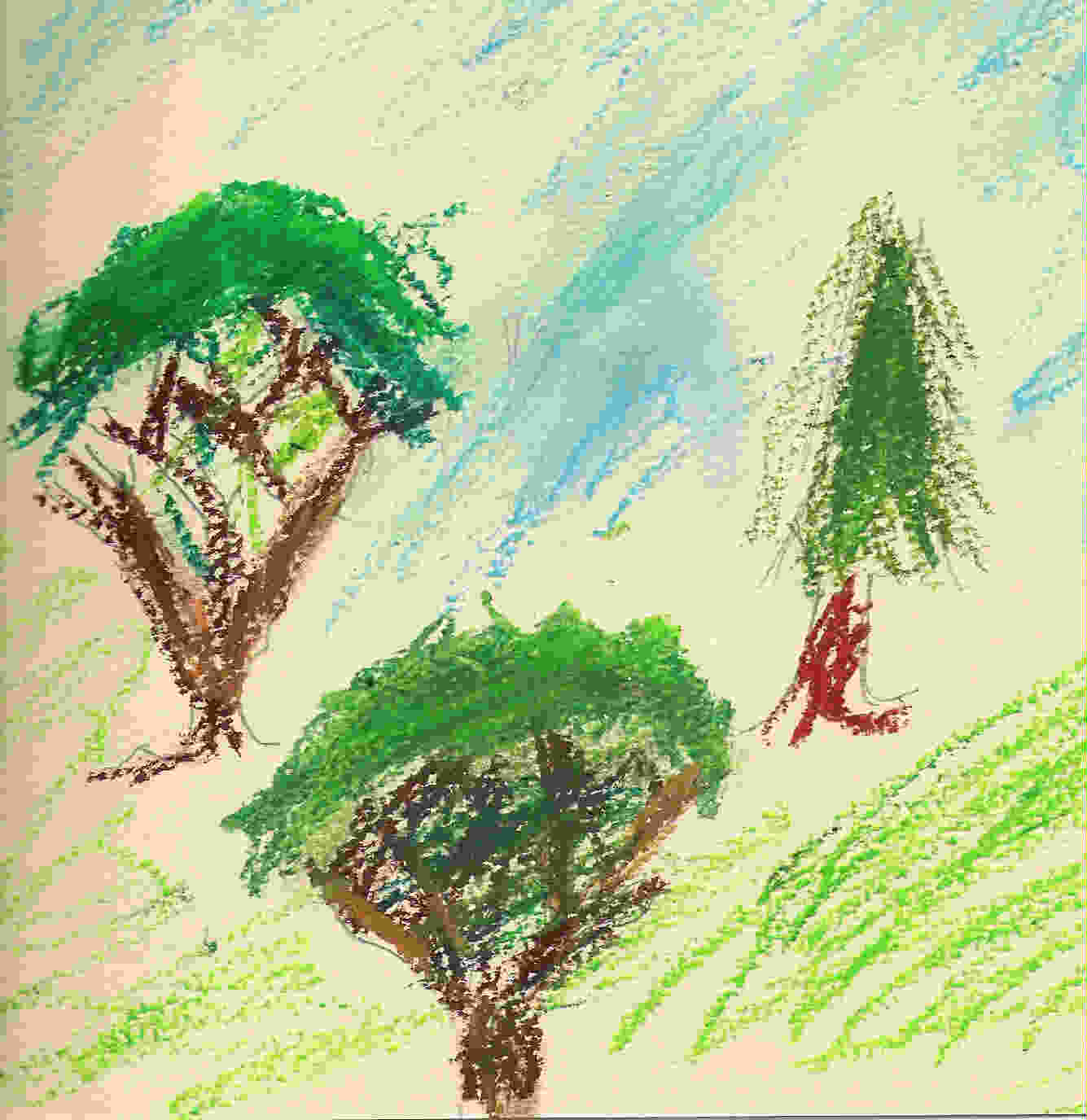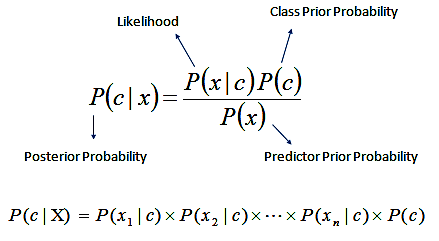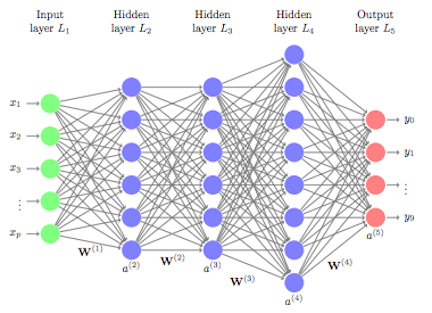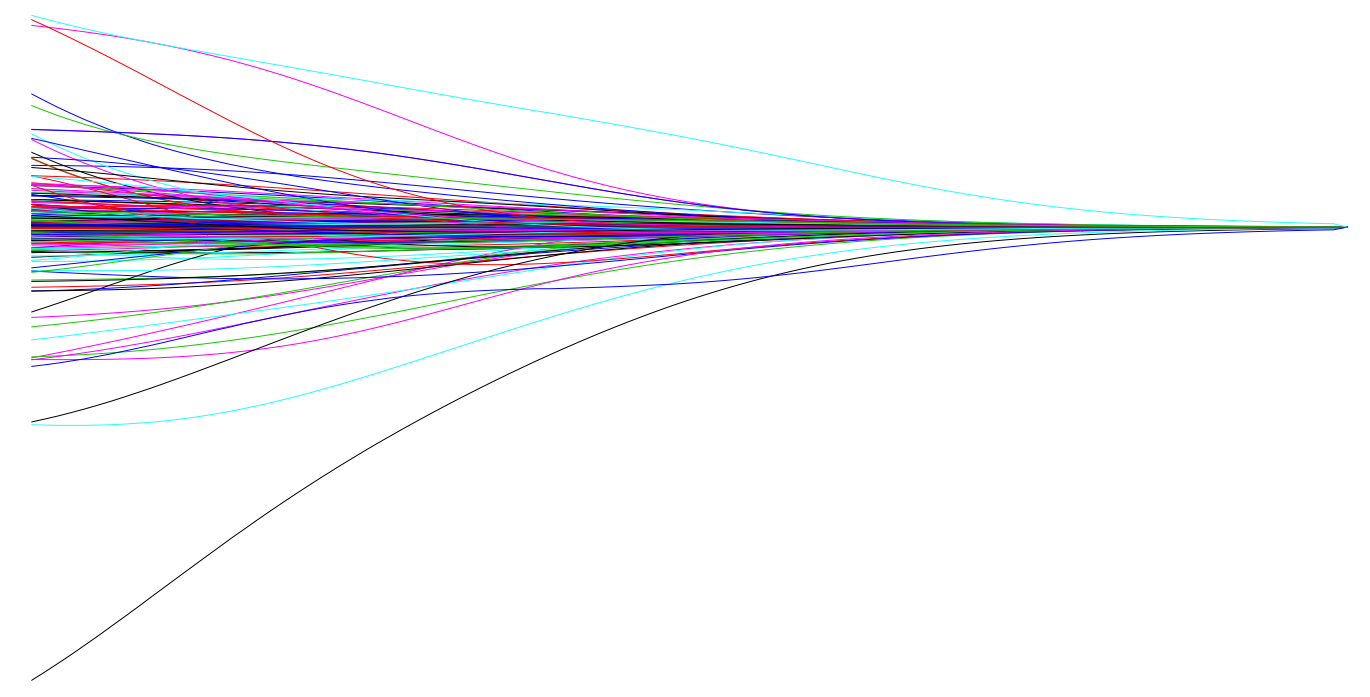# Random ForestsBagging regression trees is a technique that can turn a single tree model with high variance and poor predictive power into a fairly accurate prediction function. Unfortunately, bagging regression trees typically suffers from tree correlation, which reduces the overall performance of the model. Random forests are a modification of bagging that builds a large collection of de-correlated trees and have become a very popular “out-of-the-box” learning algorithm that enjoys good predictive performance. This latest tutorial will cover the fundamentals of random forests.

# Regression TreesBasic regression trees partition a data set into smaller groups and then fit a simple model (constant) for each subgroup. Unfortunately, a single tree model tends to be highly unstable and a poor predictor. However, by bootstrap aggregating (bagging) regression trees, this technique can become quite powerful and effective. Moreover, this provides the fundamental basis of more complex tree-based models such as random forests and gradient boosting machines. This latest tutorial will get you started with regression trees and bagging.

# Naïve Bayes ClassifierThe Naïve Bayes classifier is a simple probabilistic classifier which is based on Bayes theorem but with strong assumptions regarding independence. Historically, this technique became popular with applications in email filtering, spam detection, and document categorization. Although it is often outperformed by other techniques, and despite the naïve design and oversimplified assumptions, this classifier can perform well in many complex real-world problems. And since it is a resource efficient algorithm that is fast and scales well, it is definitely a machine learning algorithm to have in your toolkit. This tutorial will introduce you to this simple classifier.

# Feedforward Deep Learning ModelsMachine learning algorithms typically search for the optimal representation of data using some feedback signal (aka objective/loss function). However, most machine learning algorithms only have the ability to use one or two layers of data transformation to learn the output representation. As data sets continue to grow in the dimensions of the feature space, finding the optimal output representation with a shallow model is not always possible. Deep learning provides a multi-layer approach to learn data representations, typically performed with a multi-layer neural network. Like other machine learning algorithms, deep neural networks (DNN) perform learning by mapping features to targets through a process of simple data transformations and feedback signals; however, DNNs place an emphasis on learning successive layers of meaningful representations. Although an intimidating subject, the overarching concept is rather simple and has proven highly successful in predicting a wide range of problems (i.e. image classification, speech recognition, autonomous driving). This tutorial will teach you the fundamentals of building a feedfoward deep learning model.

# Regularized RegressionLinear regression is a simple and fundamental approach for supervised learning. Moreover, when the assumptions required by ordinary least squares (OLS) regression are met, the coefficients produced by OLS are unbiased and, of all unbiased linear techniques, have the lowest variance. However, in today’s world, data sets being analyzed typically have a large amount of features. As the number of features grow, our OLS assumptions typically break down and our models often overfit (aka have high variance) to the training sample, causing our out of sample error to increase. Regularization methods provide a means to control our regression coefficients, which can reduce the variance and decrease out of sample error. This latest tutorial explains why you should know this technique and how to implement it in R.